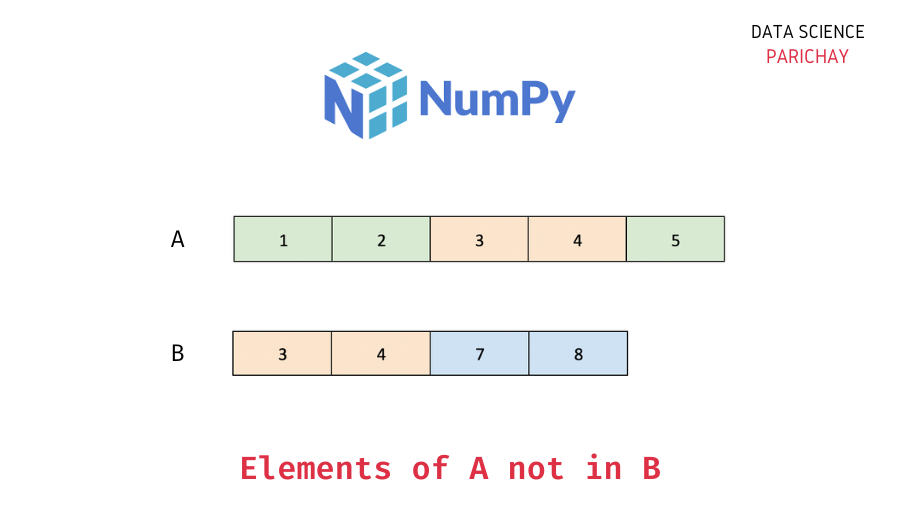# Numpy – Get Elements Not in Another Array

In this tutorial, we will look at how to get the elements of a Numpy array that are not present in another array with the help of some examples.

## How to get elements of array1 that are not in array2?

You can use a combination of the logical operator `not` and the membership operator `in` inside a list comprehension to get the elements of a Numpy array that are not present in another array.

The following is the syntax –

```# using list comprehension
[item for item in ar1 if item not in ar2]```

It results in a list of elements from the array `ar1` that are not in the array `ar2`.

## Example – Numpy Array elements not in another array

Let’s now look at some examples of using the above syntax.

Let’s create two Numpy arrays having some (but not all) elements common between them. And then use the syntax mentioned above to get elements in array1 that are not present in array2.

```import numpy as np

# create two numpy arrays
ar1 = np.array([1, 2, 3, 4, 5])
ar2 = np.array([3, 4, 7, 8])
# get elements in ar1 not in ar2
res = [item for item in ar1 if item not in ar2]
print(res)```

Output:

`[1, 2, 5]`

We get a list with elements from `ar1` that are not present in `ar2`. Now, you can convert this list to a Numpy array using the `numpy.array()` function.

📚 Data Science Programs By Skill Level

Introductory

Intermediate ⭐⭐⭐

🔎 Find Data Science Programs 👨‍💻 111,889 already enrolled

Disclaimer: Data Science Parichay is reader supported. When you purchase a course through a link on this site, we may earn a small commission at no additional cost to you. Earned commissions help support this website and its team of writers.

Also, note that elements from `ar1` that are not in `ar2` may not be the same as elements from `ar2` that are not in `ar1`.

```# get elements in ar2 not in ar1
res = [item for item in ar2 if item not in ar1]
print(res)```

Output:

`[7, 8]`

We get a list of elements of `ar2` that are not in `ar1`. You can see this result is not the same as what we got in the previous step.

The above is similar to a set difference operation. The set difference operation `a - b` gives us the elements in set a that are not in set b.

```# convert to set
s1 = set(ar1)
s2 = set(ar2)
# set difference - elements in s1 not in s2
res1 = s1 - s2
print(res1)
# set difference - elements in s2 not in s1
res1 = s2 - s1
print(res1)```

Output:

```{1, 2, 5}
{8, 7}```

You can see that `a - b` and `b - a` set operations result in different sets. For more on the set difference operation in Python, refer to this tutorial.

## Summary

In this tutorial, we looked at how we can use a combination of the logical operator `or` and the membership operator `in` inside a list comprehension to get elements of one array that are not present in another array.

You might also be interested in –

•